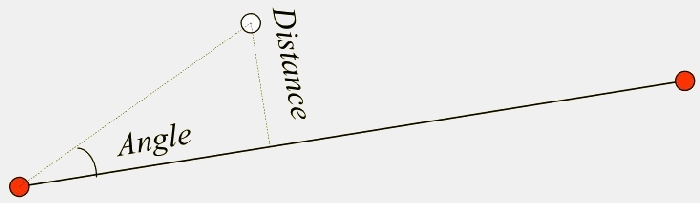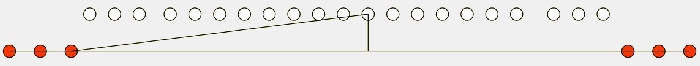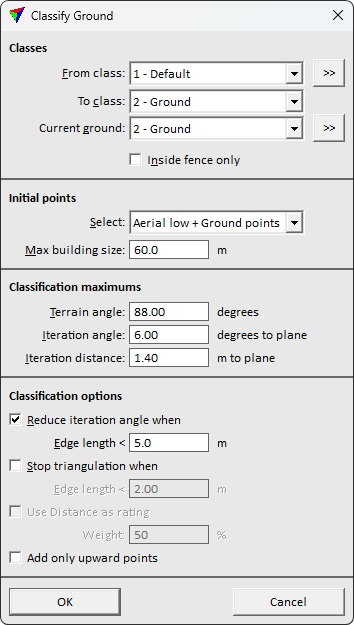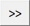# TerraScan User Guide

 Navigation: Batch Processing Reference > Classification Routines > Points Ground

Ground

Not Lite

Ground routine classifies ground points by creating a triangulated surface model iteratively. The routine is best suited for classifying ground in airborne laser data sets and in data sets where there is mainly natural terrain. For classifying ground in mobile data sets where the majority of ground is on hard surfaces, such as roads, use the Hard surface routine instead of the ground routine.

The routine is sensitive to low error points in the point cloud. Therefore, you should run one or more classification steps using the Low points routine before classifying ground. A more complex classification strategy is required for classifying ground in photogrammetric point clouds.

The ground routine starts by selecting local low points that are confident hits on the ground. The initial point selection is controlled with the Max building size parameter. If the maximum building size is, for example, set to 60.0 m, the routine assumes that any 60 by 60 m area has at least one point on the ground level and that the lowest point is on the ground level.

Then, the routine builds a surface model (TIN) from the initial ground points. The triangles in this initial model are mostly below the ground level and only the vertices are touching the ground. In the following iterations, the routine molds the model upwards by adding more and more points. Each added point makes the model following the true ground surface more closely.

The iteration parameters of the routine determine how close a point must be to a triangle plane for being accepted as ground point and added to the model. Iteration angle is the maximum angle between a point, its projection on the triangle plane and the closest triangle vertex. This is the main parameter controlling how many points are classified into the ground class. The smaller the Iteration angle, the less eager the routine is to follow variation in the ground level, such as small undulations in terrain or points on low vegetation. Use a smaller angle value (close to 4.0) in flat terrain and a bigger value (close to 10.0) in mountainous terrain.

Iteration distance makes sure that the iteration does not make big jumps upward if triangles are large. This avoids ground points that are too high, for example within low vegetation or on low buildings.Illustration of Iteration angle and Iteration distance parameters in the ground routine.A smaller Iteration distance value avoids classification of ground points on low objects.

The iteration angle can be reduced automatically, if the triangles become small. This reduces the eagerness to classify more ground points inside small triangles and thus, avoids unnecessary point density of the ground model. The iteration angle inside small triangles approaches zero if the longest triangle edge is shorter than a given Edge length value. Furthermore, the iteration can be stopped completely if triangle edges are shorter than a given limit.SETTING

EFFECT

From class

Source class(es).Opens the Select classes dialog which contains the list of active classes in TerraScan. You can select multiple source classes from the list that are then used in the From class field.

To class

Target class.

Current ground

Class(es) with already classified ground points. These points influence the classification of additional ground points but they are not effected themselves.Opens the Select classes dialog which contains the list of active classes in TerraScan. You can select multiple source classes from the list that are then used in the Current ground field.

Inside fence only

If on, points inside a fence or selected polygon(s) are classified.

Select

Selection of initial ground points. If starting a new ground classification, use Aerial low + Ground points. Use Current ground points when you want to continue the ground classification in a previously classified area.

Max building size

Defines the search area for initial ground points. The value should be close to the edge length of largest building in the project area.

Terrain angle

Steepest allowed slope in the ground surface. Use 88-90 degree if there are man-made objects in the project area. Use the estimated maximum terrain slope plus 10-15 degree if there is only natural terrain.

Iteration angle

Maximum angle between a point, its projection on the triangle plane and the closest triangle vertex.

Iteration distance

Maximum distance from a point to the triangle plane. Normally values between 0.5 and 1.5 m.

Reduce iteration angle when

If on, reduces the eagerness to add new points to ground inside a triangle if every edge of the triangle is shorter than Edge length. Avoids the addition of unnecessary point density to the ground model and reduces memory requirement.

Stop triangulation when

If on, stops processing points inside a triangle if every edge of the triangle is shorter than Edge length. Avoids the addition of unnecessary point density to the ground model and reduces memory requirement.

Use distance as rating

If on, the vegetation index stored as distance is used to determine how likely a point is to be ground. This can improve the ground classification result for photogrammetric point clouds. If used, the routine is less eager to classify points with a positive distance value (= more likely a vegetation point) and rising above other points in the local environment as ground. Points with a negative distance value (= more likely a non-vegetation point) and points in planar terrain are more easily accepted as ground points.

In a similar way, the echo length or deviation stored as distance is used to determine how likely a point is to be ground in a LiDAR point cloud. Distance values ≤0 are considered to be ground, while values >0 are less likely accepted as ground points. The closer a value to +1, the more unlikely a point is accepted to ground.

A smaller Weight value translates to a smaller effect of the setting for the ground classification, a larger value to a bigger effect.

The positive effect of the setting for classifying ground can be improved by smoothing the distance values that represent the vegetation index, echo length or deviation.

After running the automatic ground routine, there might be the need to improve the result of ground classification manually. You may use half-automatic ways in order to do this task. One possibility is to run the ground routine on loaded points and inside a fence using the Inside fence only option of the routine. Alternatively, the Add point to ground command can be used to classify more points into the ground class within a limited area.

Ground classification in photogrammetric point clouds

The ground routine often fails to detect the real ground in photogrammetric point clouds because the geometric conditions within the point cloud are different from those in LiDAR point clouds. Here it helps to use the color information in addition to point-to-point relationships. The following steps illustrate an example workflow for ground classification in photogrammetric point clouds. All steps may be also performed as macro actions.

1. Sort points by Xy location.

2. Classify major surfaces.

3. Smooth XYZ for the surface points found in step 2.

4. Thin the surface points found in step 2 by density, keep the central point within reasonable XY and Z distances (depending on the noise level in the data). The remaining points in the surface class now represent cleaner surfaces.

5. If color information is noisy, classify points that are perfectly white.

6. Classify hard surface from points that remain in the surface class after step 4. Use a planarity tolerance that allows some point variation on surfaces.

7. Compute distance for hard surface points found in step 6 by using the Visual band difference (RGB available) or the Normalized vegetation index (RGB + Infra-red available). You may check a good limit value by displaying the point cloud by vegetation index and playing with the limit setting.

8. Smooth the distance in order to reduce the noise in vegetation index values derived from color information.

9. Classify ground and make use of the Use distance as rating setting as described above.

TerraScan User Guide   26.07.2021   © 2021 Terrasolid Ltd